﻿ 人口老龄化影响财政与货币政策的有效性吗？
 财经研究2018, Vol. 44Issue (7): 16-320

#### 文章信息财经研究2018年44卷第7期

Li Jianqiang, Zhang Shucui.

Aging and the effectiveness of fiscal and monetary policies

Journal of Finance and Economics, 2018, 44(7): 16-32.

### 文章历史《财经研究》
2018第44卷第7期

1. 中国人民银行 金融研究所，北京 100800;
2. 中国电子信息产业发展研究院/赛迪研究院 工业经济研究所，北京 100846

Aging and the Effectiveness of Fiscal and Monetary Policies
Li Jianqiang1, Zhang Shucui2
1. Financial Research Institute，People’s Bank of China，Beijing 100800，China;
2. lnstitute of lndustrial Economics，Center of China Electronic Information Industry Development Institute，Beijing 100846，China
Summary: This paper introduces the population structure, the fiscal expenditure structure and the monetary policy into the new Keynesian dynamic stochastic general equilibrium framework, focusing on the analysis of the impact of population aging on China’s macro-economic control policies. The results of the study show that: In addition to the negative impact of the aging population itself on economic growth, the space for maneuvers left for macro-control policies has become increasingly limited, affecting the effectiveness of policy control and increasing the cost of policy implementation.　　First, with the aging population structure, the positive effects of macroeconomic control policies have been weakened and the negative effects have been strengthened. This shows that population aging has undoubtedly weakened the effectiveness of fiscal and monetary policies. In terms of macroeconomics, the basic effect of population aging is that it will profoundly change the relationship between the national income distribution pattern and the allocation of economic resources. In the long run, population aging reduces labor supply, and the overall economic life cycle gap widens the lack of economic growth and the overall welfare level of society continues to decline. In the short term, accelerating the burden of financial pensions has significantly reduced the space for fiscal policy maneuvers and also weakened the ability of the government to implement anti-cyclical fiscal policies. In terms of policy assessment, the decline in fiscal multipliers has masked the enthusiasm of the financial sector, amplifying its crowding-out effect and impairing the quality of financial performance. In terms of the monetary policy, population aging may bring deflationary pressures, limit the monetary policy’s ability to stimulate aggregate demands, force the Central Bank to adopt more radical measures to achieve the same effect, and be directly exposed to higher macro-economic risk exposures and strengthen financial fragility. 　　Second, in the elderly-dominated society, the central bank’s tolerance for inflation rate has decreased, and interest rate adjustments are more likely to approach the lower limit. Once these new weighing factors are superimposed on the cycle, they will further complicate the operation of the monetary policy. The Central Bank must not only prevent the spread of inflation, protect the economic interests of the elderly, but also prevent the " hard landing” economic risks caused by excessive tightening. Objectively, the frequency of policy switching will exceed the previous level, and unconventional monetary policies will probably become the norm. In terms of stabilizing the economy and finance, the lack of effective demand caused by population aging has weakened the effectiveness of traditional monetary policy operations. Severe aging may further trigger liquidity traps and no longer be affected by loose monetary policies. Low interest rates are stimulating investment and consumption. The role is limited. Enterprises no longer pursue profit maximization but minimize debt. As a result, the demand for bank credit is insufficient and the liquidity of the central bank is difficult to release. Under such circumstances, structural reform will become a necessary supplement to the monetary policy.　　Third, increasing the proportion of working population and maximizing the negative effects of accelerating population aging can greatly improve and increase the effectiveness of fiscal and monetary policies. The loose population policy does not necessarily change the demographic structure and promote economic growth in the short term. The economic problems brought about by population aging are a rather complicated social project. It is not simply a matter of " opening up policies and stimulating fertility” that can open the door to alleviate the aging population. Moreover, increasing the labor participation rate will increase the proportion of the working population to a certain extent, effectively increase the supply of labor force, and reduce the tax burden on each of the young people. More importantly, increasing the labor participation rate can effectively increase the labor supply, not only helping to ease the burden of government pensions and guaranteeing the reliability of the pension system in the medium term, but also helping to reduce macroeconomic fluctuations, expand fiscal and monetary policies to create more space for operations, stimulate private consumption and investment, and drive economic growth.
Key words: policy effects    fiscal multiplier    frontier curve图 1 模型结构

（一）家庭部门

 ${E_0}\sum\limits_{t = 0}^\infty {{\beta ^t}} \left\{ {\frac{1}{{1 - \sigma }}{{\left[ {{{\left\{ {\omega c_{w,t}^{\frac{{\zeta - 1}}{\zeta }} + (1 - \omega )G_t^{\frac{{\zeta - 1}}{\zeta }}} \right\}}^{\frac{\zeta }{{\zeta - 1}}}}} \right]}^{1 - \sigma }} - \frac{{l_{w,t}^{1 + \mu }}}{{1 + \mu }}} \right\}$ (1)

 ${c_{w,t}} + {b_{w,t}} + {T_{w,t}} = {w_t}{l_{w,t}} + \frac{{R_{t - 1}^d{b_{w,t - 1}}}}{{{\pi _t}}} + {\Pi _{w,t}}$ (2)

 ${E_0}\sum\limits_{t = 0}^\infty {{\beta ^t}} \left\{ {\frac{1}{{1 - \sigma }}{{\left[ {{{\left\{ {\omega c_{r,t}^{\frac{{\zeta - 1}}{\zeta }} + (1 - \omega )G_t^{\frac{{\zeta - 1}}{\zeta }}} \right\}}^{\frac{\zeta }{{\zeta - 1}}}}} \right]}^{1 - \sigma }}} \right\}$ (3)

 ${c_{r,t}} + {b_{r,t}} = \frac{{R_{t - 1}^d{b_{r,t - 1}}}}{{{\pi _t}}} + {s_t}$ (4)

（二）厂商部门

 ${Y_{j,t}} = {\left( {\frac{{{P_{j,t}}}}{{{P_t}}}} \right)^{ - \varepsilon }}{Y_t}$ (5)

 $\begin{array}{l}{\rm{Min}}{E_0}\sum\nolimits_{t = 0}^\infty {{\gamma ^t}{\Lambda _t}{\Psi _t}} \\{\rm{S}}.{\rm{t}}\;{\Psi _t} = {w_t}{L_t} + {I_t} + \frac{{R_{t - 1}^l{B_{t - 1}}}}{{{\pi _t}}} - {B_t}\end{array}$ (6)
 ${K_t} = (1 - \delta ){K_{t - 1}} + {I_t}$ (7)
 ${Y_{j,t}} = {A_t}{({K_{j,t - 1}})^\alpha }{({L_{j,t}})^{1 - \alpha }}K_{g,t - 1}^{{\alpha _g}}$ (8)

 ${w_t} = (1 - \alpha )m{c_t}\frac{{{Y_{j,t}}}}{{{L_{j,t}}}}$ (9)
 $\frac{{R_t^l}}{{{\pi _{t + 1}}}} = \alpha m{c_t}\frac{{{Y_{j,t + 1}}}}{{{K_{j,t}}}} + 1 - \delta$ (10)
 $\frac{{R_t^l}}{{{\pi _{t + 1}}}} = \frac{{{\Lambda _t}}}{{\gamma {\Lambda _{t + 1}}}}$ (11)

 $P_{j,t}^* = \frac{\varepsilon }{{\varepsilon - 1}}\frac{{{E_t}\sum\nolimits_{i = 0}^\infty {{{(\beta \gamma \xi )}^i}{\Lambda _{t + i}}m{c_{t + i}}{Y_{t + i}}} }}{{{E_t}\sum\nolimits_{i = 0}^\infty {{{(\beta \gamma \xi )}^i}{\Lambda _{t + i}}\frac{{{Y_{t + i}}}}{{{P_{t + i}}}}} }}$ (12)

 ${P_t} = {[\zeta P_{t - 1}^{1 - \varepsilon } + (1 - \zeta )P_{j,t}^{*1 - \varepsilon }]^{\frac{1}{{1 - \varepsilon }}}}$ (13)

（三）金融市场

 $(1 - \varphi )R_t^l = R_t^d$ (14)

（四）政府部门

 $\frac{{R_t^d}}{{{R^d}}} = {\left( {\frac{{R_{t - 1}^d}}{{{R^d}}}} \right)^{{\rho _R}}}{\left[ {{{\left( {\frac{{{\pi _t}}}{\pi }} \right)}^{{\varphi _\pi }}}{{\left( {\frac{{{Y_t}}}{Y}} \right)}^{{\varphi _Y}}}} \right]^{1 - {\rho _R}}}{e^{{u_{Rd,t}}}}$ (15)

 $(1 - \varphi ){T_{w,t}} = {G_t} + {I_{g,t}} + \varphi {s_t}$ (16)

 ${K_{g,t}} = (1 - {\delta _g}){K_{g,t - 1}} + {I_{g,t}}$ (17)

 $\frac{{{I_{g,t}}}}{{{I_g}}} = {\left( {\frac{{{I_{g,t - 1}}}}{{{I_g}}}} \right)^{{\rho _{ig}}}}{\left[ {{{\left( {\frac{{{Y_t}}}{Y}} \right)}^{{\varphi _{ig}}}}} \right]^{1 - {\rho _{ig}}}}{e^{{u_{Ig,t}}}}$ (18)
 $\frac{{{G_t}}}{G} = {\left( {\frac{{{G_{t - 1}}}}{G}} \right)^{{\rho _g}}}{\left[ {{{\left( {\frac{{{Y_t}}}{Y}} \right)}^{{\varphi _g}}}} \right]^{1 - {\rho _g}}}{e^{{u_{G,t}}}}$ (19)

（一）参数校准

 参数 经济含义 取值 参数 经济含义 取值 ${\beta }$ 居民贴现率 0.99 ${\sigma }$ 风险厌恶系数 1 ${\mu }$ Frisch弹性的逆 2 ${\xi }$ 私人消费与财政消费替代弹性 0.4 ${\omega }$ 消费品权重 0.4 ${{\alpha _g}}$ 财政投资产出弹性 0.06 ${\alpha }$ 资本份额 0.45 ${\delta }$ 私人资本折旧率 0.025 ${{\delta _g}}$ 公共资本折旧率 0.05 ${\varepsilon }$ 商品替代弹性 11 ${\zeta }$ Calvo价格粘性 0.7 ${\varphi }$ 老年人口占比 9.58% ${{\varphi _\pi }}$ 通胀反馈系数 1.5 ${\psi }$ 工资替代率 0.6 ${{\varphi _Y}}$ 产出缺口反馈系数 0.125 ${{\varphi _{ig}}}$ 财政投资内生反馈系数 −0.4 ${{\varphi _g}}$ 财政消费内生反馈系数 −0.6 ${{\rho _R}}$ 利率平滑可持续性 0.9 ${{\rho _g}}$ 财政消费支出可持续性 0.9 ${{\rho _{ig}}}$ 财政投资支出可持续性 0.9 ${\rho _{Rd}^u}$ 利率外生冲击自回归系数 0.5 ${{\rho ^u}}$ 财政支出外生冲击自回归系数 0.7 Ig/Y 财政投资性支出占GDP比重 0.059 G/Y 财政消费支出占GDP比重 0.128

（二）长期稳态分析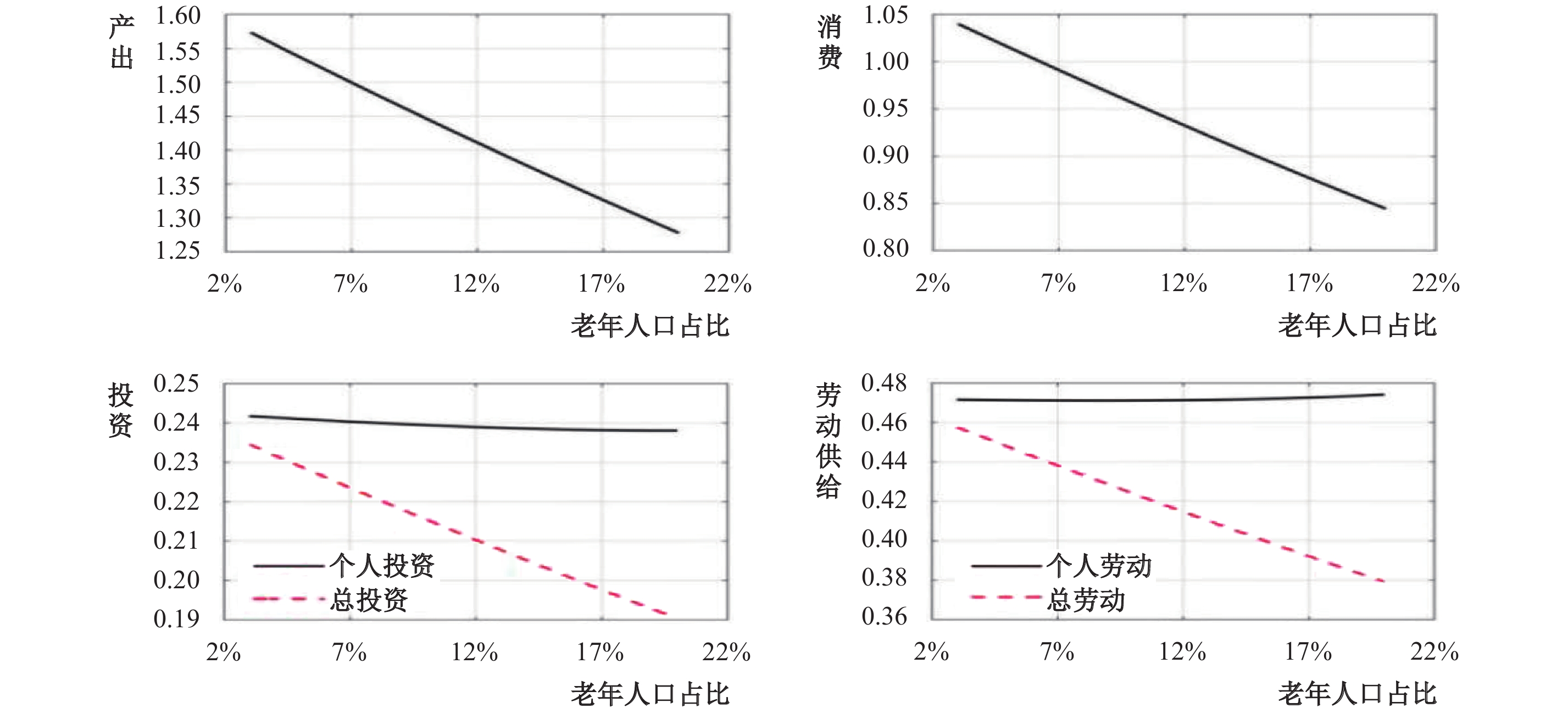图 2 人口老龄化对宏观经济的影响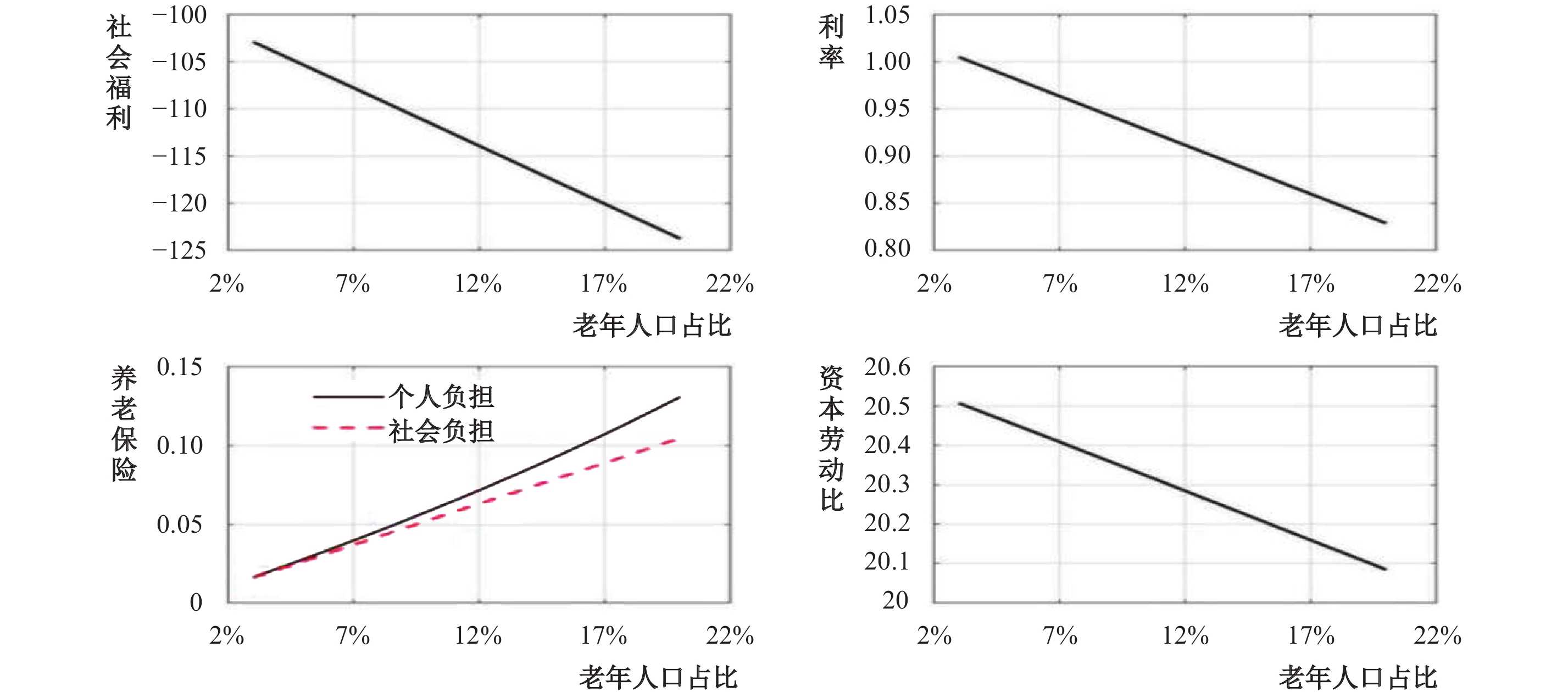图 3 人口老龄化对社会福利的影响

（三）短期动态分析

1. 财政支出的脉冲响应分析。人口总体结构改变不仅影响长期经济稳态，对短期财政刺激效果同样具有决定性影响。为进一步解释不同人口结构下财政支出冲击的传导机制和动态特征，本文将老年人口占比作为人口老龄化指标，分别模拟比较了老龄化、深度老龄化和超老龄化三个阶段的影响作用。图 4 财政消费支出冲击的经济效应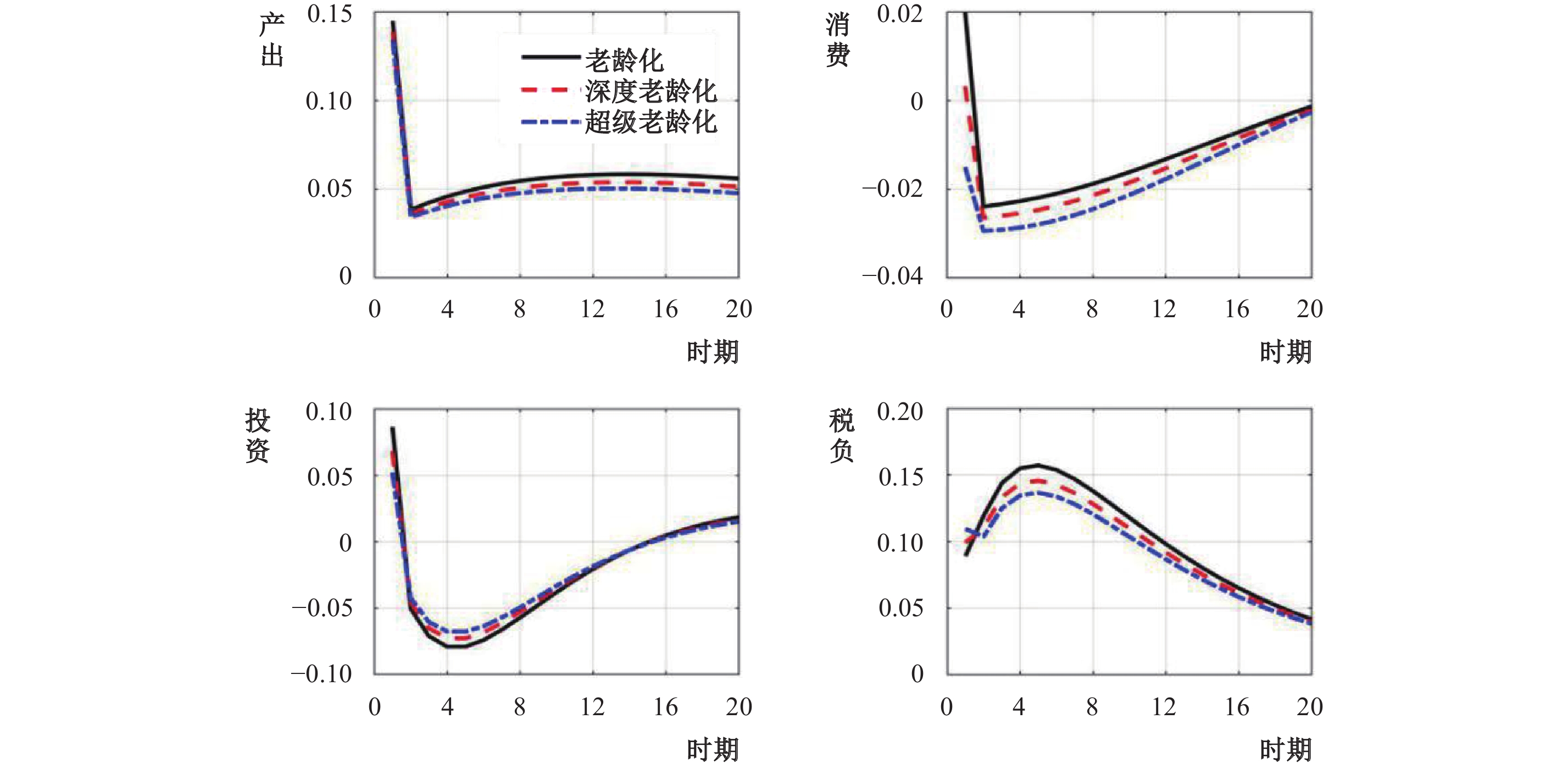图 5 财政投资支出冲击的经济效应

2. 财政乘数效应分析。财政乘数表示一单位财政支出引起多少单位GDP的增加，这是衡量财政刺激效应大小的一个重要指标。根据定义，我们可分别计算长期财政乘数和短期财政乘数。所谓长期财政乘数是指稳态时的财政乘数，而短期财政乘数则是指不同时期内的财政乘数。复杂的模型往往得不到财政乘数的解析表达式，为揭示人口结构与财政乘数之间的关系，我们对模型的生产函数进行简化，并定义稳态财政乘数为：

 $\overline {Mul} = \frac{{\Delta \overline Y }}{{\Delta \overline G }}$

 ${Y^\mu } = \frac{{{A^{1 + \mu }}{{({I_g}/{\delta _g})}^{{\alpha _g}(1 + \mu )}}(1 - \varphi )}}{{(1 + \displaystyle\frac{{\varphi \psi mc}}{{1 - \varphi }})Y - {I_g}}}$ (20)

$1 + \varphi \psi mc/(1 - \varphi ) = M$ $mc$ 是边际成本，（20）式两边同时对财政投资求导得：

 $[M(1 + \mu ){Y^\mu } - \mu {Y^{\mu - 1}}{I_g}]dY/d{I_g} - {Y^\mu } = (1 - \varphi )/\delta _g^{{\alpha _g}(1 + \mu )}{\alpha _g}(1 + \mu )I_g^{{\alpha _g}(1 + \mu ) - 1}$

（22）式两边同乘财政投资后，将（20）式代入右边可得：

 $[M(1 + \mu ){Y^\mu } - \mu {Y^{\mu - 1}}{I_g}]dY/d{I_g}{I_g} - {Y^\mu }{I_g} = {\alpha _g}(1 + \mu )(M{Y^{1 + \mu }} - {Y^\mu }{I_g})$

（23）式两边同除 ${Y^\mu }{I_g}$ 化简可得：

 $dY/d{I_g} = \frac{{1 + {\alpha _g}(1 + \mu )(M/{s_{Ig}} - 1)}}{{M(1 + \mu ) - \mu {s_{Ig}}}}$ (21)

 $\frac{\partial }{{\partial M}}\left( {\frac{{dY}}{{d{I_g}}}} \right) = \frac{{(1 + \mu ){\alpha _g}}}{{{{[(1 + \mu )M - \mu {s_{Ig}}]}^2}}}$

$M$ 关于人口老龄化 $\varphi$ 的偏导可得：

 $\frac{{\partial M}}{{\partial \varphi }} = \frac{{\psi mc}}{{{{(1 - \varphi )}^2}}}$

 $\frac{\partial }{{\partial \varphi }}\left( {\frac{{dY}}{{d{I_g}}}} \right) = \frac{\partial }{{\partial M}}\left( {\frac{{dY}}{{d{I_g}}}} \right) \frac{{\partial M}}{{\partial \varphi }} = \frac{{(1 + \mu )({\alpha _g} - 1)}}{{{{[(1 + \mu )M - \mu {s_{Ig}}]}^2}}} \frac{{\psi mc}}{{{{(1 - \varphi )}^2}}} < 0$ (22)

 ${Y^\mu } = \frac{{{A^{1 + \mu }}\omega (1 - \varphi )mc}}{{N\left( {(1 + \displaystyle\frac{{\varphi \psi mc}}{{1 - \varphi }})Y - G} \right)}}$ (23)

 $NG\mu {Y^{\mu - 1}}dY/dG - M(1 + \mu )N{Y^\mu }dY/dG - N{Y^\mu } = 0$

（29）式两边同除 $N{Y^\mu }$ 化简可得：

 $dY/dG = \frac{1}{{M(1 + \mu ) - \mu {s_g}}}$ (24)

 $\frac{\partial }{{\partial \varphi }}\left( {\frac{{dY}}{{dG}}} \right) = \frac{\partial }{{\partial M}}\left( {\frac{{dY}}{{dG}}} \right) \frac{{\partial M}}{{\partial \varphi }} = \frac{{ - (1 + \mu )}}{{{{[(1 + \mu )M - \mu {s_{Ig}}]}^2}}} \frac{{\psi mc}}{{{{(1 - \varphi )}^2}}} < 0$ (25)

（31）式表明人口老龄化与财政消费乘数成负相关关系，这意味着随着人口老龄化的加重，长期财政消费乘数效应逐渐减弱。关于短期财政乘数，我们采用两种方法，一是在一定时期内衡量累积的刺激效应，可表示为：

 $Mul_t^{cum} = \frac{{\sum\limits_{i = 0}^K {\Delta {Y_{t + i}}} }}{{\sum\limits_{i = 0}^K {\Delta {G_{t + i}}} }}$

 $Mul_t^{pv} = \frac{{\sum\limits_{i = 0}^K {{{(1 + r)}^{ - i}}\Delta {Y_{t + i}}} }}{{\sum\limits_{i = 0}^K {{{(1 + r)}^{ - i}}\Delta {G_{t + i}}} }}$

 财政投资支出 财政消费支出 老龄化 ${\varphi = 7\% }$ 深度老龄化 ${\varphi = 14\% }$ 超级老龄化 ${\varphi = 20\% }$ 老龄化 ${\varphi = 7\% }$ 深度老龄化 ${\varphi = 14\% }$ 超级老龄化 ${\varphi = 20\% }$ 累积财政乘数 ${Mul_t^{cum}}$ 1个季度 2.734 2.714 2.692 2.034 1.957 1.885 2个季度 1.640 1.630 1.129 1.170 1.133 1.098 4个季度 1.121 1.116 0.987 1.034 1.015 0.082 8个季度 0.092 0.089 0.087 0.081 0.072 0.065 折现累积财政乘数 ${Mul_t^{pv}}$ 1个季度 2.734 2.714 2.692 2.034 1.957 1.885 2个季度 1.683 1.681 1.181 1.205 1.166 1.130 4个季度 1.174 1.168 0.997 1.039 1.022 0.090 8个季度 0.095 0.093 0.092 0.089 0.083 0.078

3. 货币政策的脉冲响应分析。图6显示，人口老龄化并没有实质性扭转货币政策的经济效应，但确实在一定程度上弱化了其对宏观经济的影响力。当中央银行采取宽松货币政策，即降低名义利率时，原本通过实际利率下降，促进消费和投资需求上升，拉动总需求增加，继而带动工资和劳动供给增加，提高产出和总收入。但是，在不同程度的老龄化水平下，实际利率下降幅度有所区别，社会融资成本有差异，投资需求相应增加的幅度也不同。这说明在人口老龄化影响下，央行通过旨在控制通胀预期以减少左右投资决策和抑制消费不确定性、并对外部冲击做出灵活响应的这种作用被弱化。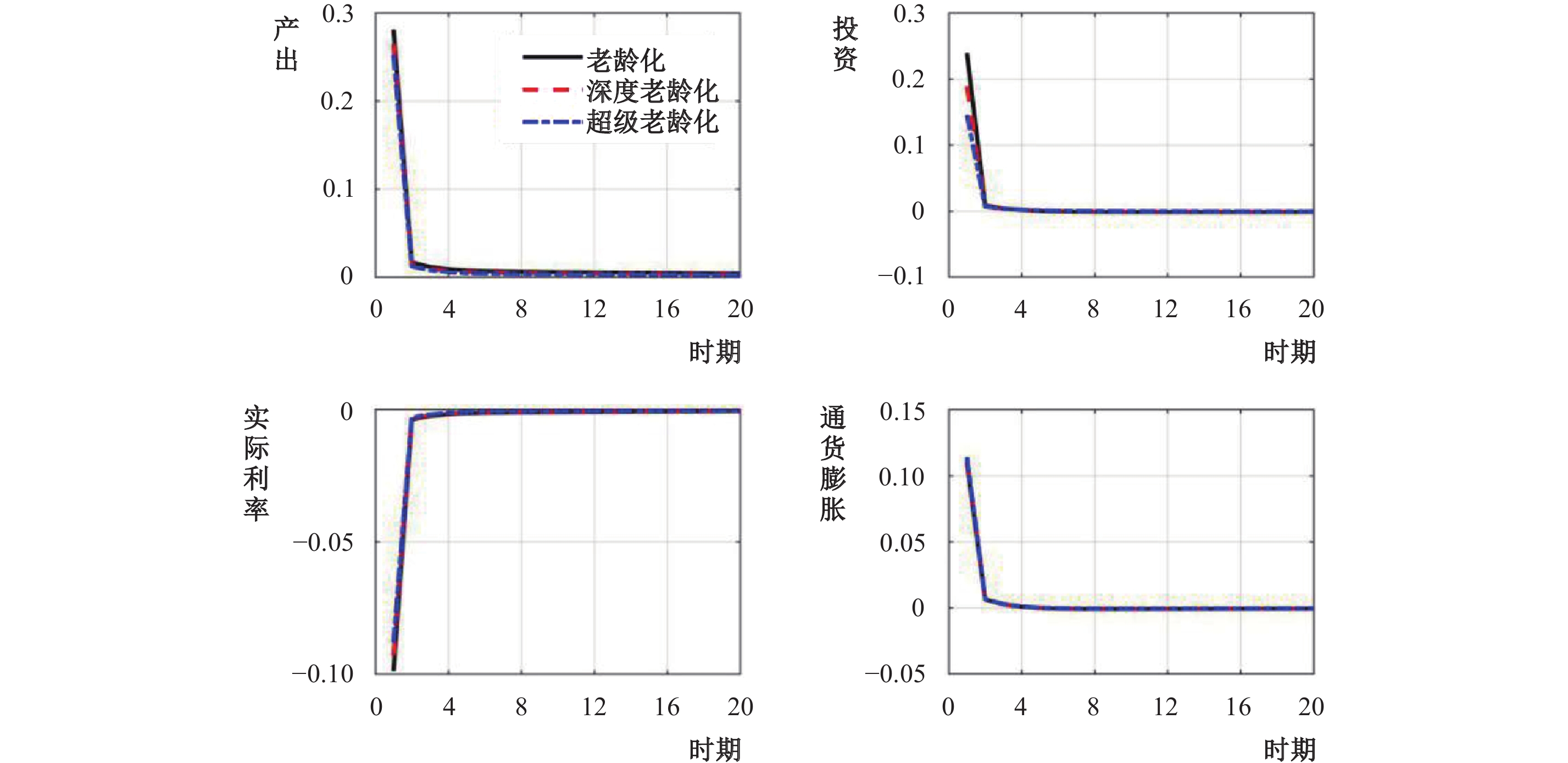图 6 货币政策冲击的经济效应

4. 政策前沿曲线分析。除了人口老龄化对货币政策实施效果的影响外，随着时间的推移，缓慢变化的人口因素在中央银行的最优规则制定过程中会日益凸显出来。为了刻画这种影响，本文采用政策前沿曲线进行分析。给定中央银行的目标函数是二次损失函数（Woodford，2003），使产出和通货膨胀波动率的加权平均最小化。对数线性化后的模型可表示为货币政策参数 ${\varphi _\pi }$ ${\varphi _Y}$ 的函数，在给定权重下搜索政策参数空间以使损失函数值最小，进而求得相应的通胀和产出波动率组合。当权重发生连续变化时，这些点的组合就构成了最优货币政策规则下的政策前沿曲线（Schmitt和Uribe，2007）。

 ${\rm{Min}}L = \lambda \operatorname{var} ({y_t}) + (1 - \lambda )\operatorname{var} ({\pi _t})$
 ${\rm{S}}{\rm{.t}}\;{x_t} = \Gamma ({\varphi _\pi },{\varphi _Y}){x_{t - 1}} + \Omega ({\varphi _\pi },{\varphi _Y}){\varepsilon _t}$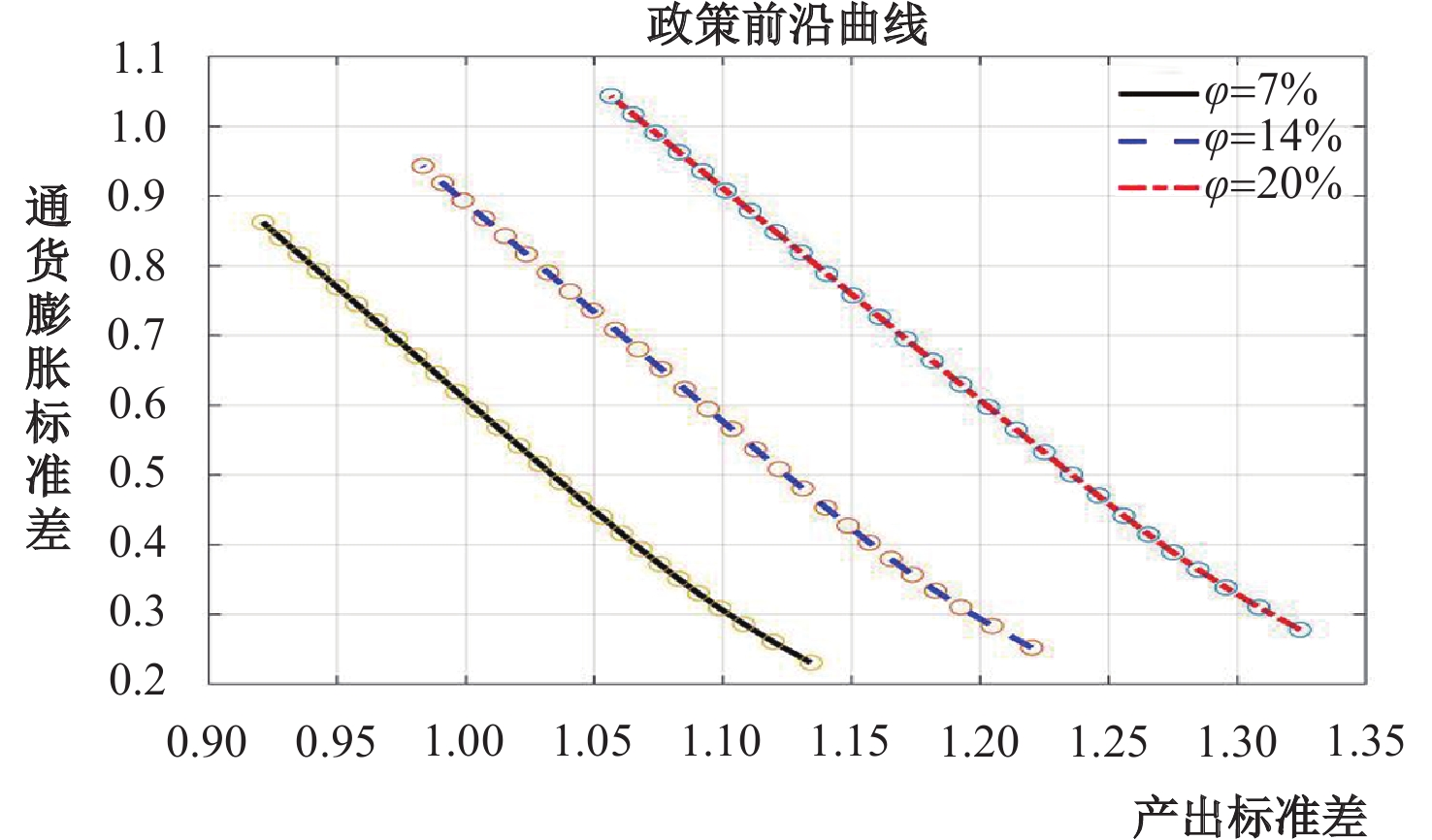图 7 政策前沿曲线

（四）动态转移分析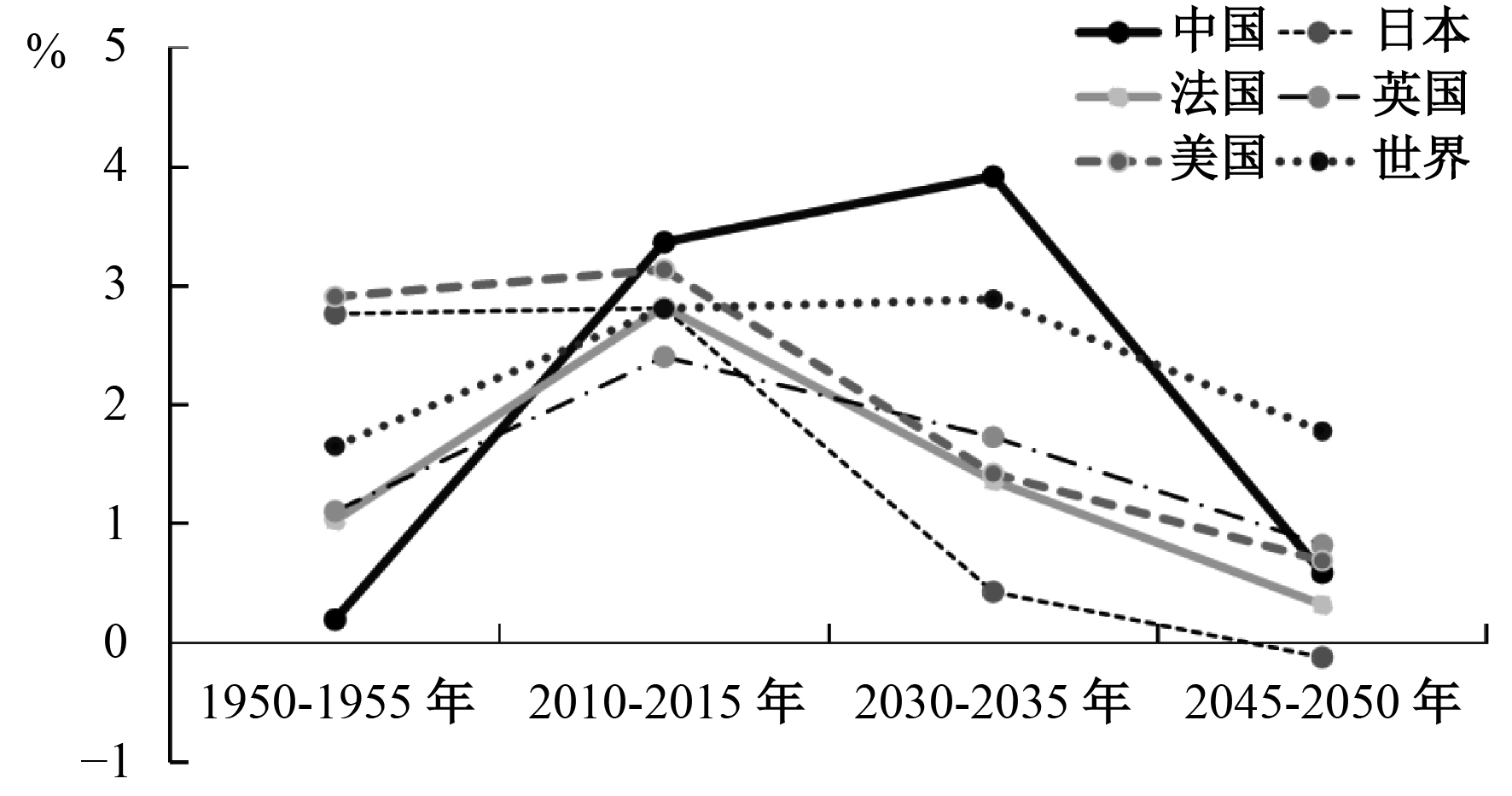图 8 主要国家人口老龄化速度 数据来源：联合国人口和社会统计。图 9 人口老龄化速度对经济的影响

① 考虑到收入流动性的限制，他们将居民分为李嘉图与非李嘉图经济主体两类，同理考虑到人口年龄结构的差异，我们将居民分为年轻人与老年人两类。

② 定义社会福利函数为 $W = \phi {W_w} + (1 - \phi ){W_r}$ ，这里 ${W_w}$ ${W_r}$ 分别表示年轻人和老年人的效用水平。

③ 央行通过盯住损失函数，旨在控制通胀预期，减少了左右投资决策和抑制消费的不确定性，并能够对冲击做出灵活响应。

④ 研究显示，随着众多选民的老龄化，一国决策将更多地体现老年人的意识和关注老年人的福利（Ali，2008）。

  巴曙松. 人口结构的宏观金融含义[R]. 中国金融四十人论坛报告, 2012.  蔡昉. 人口转变、人口红利与刘易斯转折点[J]. 经济研究, 2010(4): 4–13.  陈小亮, 马啸. " 债务-通缩”风险与货币政策财政政策协调[J]. 经济研究, 2016(8): 28–41.  都阳. 人口转变、劳动力市场转折与经济发展[J]. 国际经济评论, 2010(6): 136–148.  龚锋, 余锦亮. 人口老龄化、税收负担与财政可持续性[J]. 经济研究, 2015(8): 16–30.  郭长林, 胡永刚, 李艳鹤. 财政政策扩张、偿债方式与居民消费[J]. 管理世界, 2013(2): 64–77.  黄志刚. 价格非平衡变化的机理: 一个新凯恩斯主义的解释[J]. 南方经济, 2010(4): 52–64.  李时宇, 冯俊新. 老龄化对中国政府资产负债表影响及政策空间比较分析[J]. 经济学动态, 2014(1): 78–81.  刘穷志, 何奇. 人口老龄化、经济增长与财政政策[J]. 经济学, 2013(1): 119–134.  刘枭, 李雪, 郑棣. 人口老龄化对我国货币政策传导机制的影响[J]. 财经科学, 2014(9): 41–48.  刘学良. 中国养老保险的收支缺口和可持续性研究[J]. 中国工业经济, 2014(9): 25–37.  罗纳德·李, 安德鲁·梅森. 老龄化的成本[J]. 金融与发展, 2017(3): 7–9.  马轶群, 任媛. 中国人口老龄化冲击下出生率变动的经济效果[J]. 人口与经济, 2017(2): 21–31.  帕特里克. 老龄化危机[J]. 世界银行金融与发展, 2014(3): 20–24.  彭文生. 渐行渐远的红利: 寻找中国新平衡[M]. 北京: 社会科学文献出版社, 2013.  王国静, 田国强. 政府支出乘数[J]. 经济研究, 2014(9): 4–19.  王小鲁, 樊纲. 中国经济增长的可持续性——跨世纪的回顾与展望[M]. 北京: 经济科学出版社, 2000.  汪伟, 艾春荣. 人口老龄化与中国储蓄率的动态演化[J]. 管理世界, 2015(6): 47–62.  伍戈, 曾庆同. 人口老龄化和货币政策: 争议与共识[J]. 国际经济评论, 2015(4): 99–109.  许志伟, 林仁文. 我国总量生产函数的贝叶斯估计——基于动态随机一般均衡的视角[J]. 世界经济文汇, 2011(2): 87–102.  薛鹤翔. 中国的产出持续性——基于刚性价格和刚性工资模型的动态分析[J]. 经济学（季刊）, 2010(4): 1359–1384.  张军, 章元. 对中国资本存量K的再估计[J]. 经济研究, 2003(7): 35–43.  周源, 唐晓婕. 人口老龄化与货币政策有效性[J]. 西安交通大学学报（社会科学版）, 2015(5): 25–32.  Andersen T M. Fiscal sustainability and fiscal policy targets[R]. ASB Economic Working Paper, 2012.  Aschauer D. Fiscal policy and aggregate demand[J]. American Economic Review, 1985, 75(1): 117–270.  Barro R. Government spending in a simple model of endogenous growth[J]. Journal of Political Economy, 1990, 98(5): 103–126. DOI:10.1086/261726  Bean C. Global demographic change: Some implications for central banks[R]. Bank of England Working Paper, 2004.  Bloom D E, Canning D, Fink G. Implications of population aging for economic growth[R]. NBER Working Paper No. 16705, 2011.  Borio C, Zhu H. Capital regulating, risk-taking and monetary policy: A missing link in the transmission mechanism[R]. BIS Working Papers No. 268, 2008.  Bruckner M, Pappa E. Fiscal expansions, unemployment, and labor force participation: Theory and evidence[J]. International Economic Review, 2012, 53(4): 1205–1228. DOI:10.1111/j.1468-2354.2012.00717.x  Burtless G. The impact of population aging and delayed retirement on workforce productivity[R]. Center for Retirement Research at Boston College, 2013.  Campbell J, Mankiw G. Consumption, income,and interest rates: Reinterpreting the time series evidence[J]. NBER Macroeconomics Annual, 1989(4): 185–216.  Cipriani G P. Population aging and PAYG pensions in the OLG model[J]. Journal of Population Economics, 2014, 27(1): 251–256. DOI:10.1007/s00148-013-0465-9  Esteban-Pretel J, Sawada Y. On the role of policy interventions in structural change and economic development:The case of postwar Japan[J]. Journal of Economic Dynamics and Control, 2014, 40(1): 67–83.  Ewijk C. Ageing and the sustainability of Dutch public finances[R]. CPB Netherlands Bureau for Economic Policy Analysis, 2006.  Fujiwara I, Hara N, Hirakata N, et al. Japanese monetary policy during the collapse of the bubble economy[J]. Monetary and Economic Studies, 2007, 28(2): 89–128.  Fujiwara I, Teranishi Y. A dynamic new Keynesian life-cycle model: Societal aging, demographics, and monetary policy[J]. Journal of Economic Dynamics and Control, 2008, 32(8): 2398–2427. DOI:10.1016/j.jedc.2007.09.002  Gali J. Monetary policy, inflation,and the business cycle[M]. Princeton: Princeton University Press, 2008.  Gertler M. Government debt and social security in a life-cycle economy[J]. Carnegie-Rochester Conference Series on Public Policy, 1999, 50: 61–110. DOI:10.1016/S0167-2231(99)00022-6  Gertler M, Karadi P. A model of unconventional monetary policy[J]. Journal of Monetary Economics, 2011, 58(1): 17–34. DOI:10.1016/j.jmoneco.2010.10.004  Hamori S, Asako K. Government consumption and fiscal policy: Some evidence from Japan[J]. Applied Economic Letters, 1999, 6(9): 551–555. DOI:10.1080/135048599352583  Imam P A. Shock from graying: Is the demographic shift weakening monetary policy effectiveness[R]. IMF Working Paper No. 13/191, 2013.  Kantur Z. Aging and monetary policy[R]. Department of Economics, Bilkent University, Ankara, Turkey, 2013.  Kara E, von Thadden L. Interest rate effects of demographic changes in a New-Keynesian life-cycle framework[R]. ECB Working Paper No. 1273, 2010.  Linnemann L, Schabert A. Fiscal policy in the new neoclassical synthesis[J]. Journal of Money, Credit, and Banking, 2003, 35(6): 911–929.  Liu Z, Miao J, Tao Z. Land prices and unemployment[J]. Journal of Money Economics, 2016(80): 86–105.  Mayer E, Moyen S, Stähler N. Government expenditures and unemployment: A DSGE perspective[R]. Deutsche Bundesbank Discussion Paper, 2010.  Mitchell P R, Sault J E, Wallis K F. Fiscal policy rules in macroeconomic models: Principles and practice[J]. Economic Modelling, 2000, 17(2): 171–193. DOI:10.1016/S0264-9993(99)00026-7  Mountford A, Uhlig H. What are the effects of fiscal policy shocks[J]. Journal of Applied Econometric, 2009, 24(6): 960–992. DOI:10.1002/jae.1079  Nakahigashi M, Yoshino N. Changes in economic effect of infrastructure and financing methods: The Japanese case[J]. Public Policy Review, 2017, 12: 47–65.  Perkins D. Reforming China’s economy system[J]. Journal of Economic Literature, 1998(2): 601–645.  Poterba J M. Demographic structure and asset returns[J]. Review of Economics and Statistics, 2001, 83(4): 565–584. DOI:10.1162/003465301753237650  Poterba J M. Population aging and financial markets[R]. NBER Working Paper, 2004.  Ripatti A. " A dynamic new Keynesian life-cycle model: Societal aging, demographics, and monetary policy” by Ippei Fujiwara and Yuki Teranishi. A comment[J]. Journal of Economic Dynamics and Control, 2008, 32(8): 2507–2511. DOI:10.1016/j.jedc.2007.09.005  Puhakka M. The effects of aging population on the sustainability of fiscal policy[R]. Bank of Finland Research Discussion Paper No. 26, 2005.  Schmitt-Grohé S, Uribe M. Optimal simple and implementable monetary and fiscal rules[J]. Journal of Monetary Economics, 2007, 54(6): 1702–1725. DOI:10.1016/j.jmoneco.2006.07.002  Thøgersen J. Population ageing and capital accumulation: A simple OLG model with PAYGO pensions[J]. Theoretical Economics Letters, 2015, 5(2): 55031.  Woodford M. Interest and prices: Foundations of a theory of monetary policy[M]. Princeton: Princeton University Press, 2003.  Yoshino N, Miyamoto H. Decreased effectiveness of fiscal and monetary policies in Japan’s aging society[R]. ADBI Working Paper, 2017.